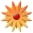# Solving 2-step Inequalities

Page Views: 1245

Email This Lesson Plan to Me
Overall Rating:(5.0 stars, 1 ratings)

 Keywords: solving/graphing inequalities, math Subject(s): Math Grades 7 through 8 NETS-S Standard: Critical Thinking, Problem Solving, and Decision MakingTechnology Operations and ConceptsView Full Text of Standards School: Oak Grove Middle School, Oak Grove, MO Planned By: Sheryl Reigel Original Author: Sheryl Reigel, Oak Grove
Solving Two-Step Inequalities
Essential Question: How can you use an inequality to describe the dimension of a figure?
Objective: Students will solve and graph two-step inequalities.
Previous Learning: Students should know how to solve two-step equations.

CC State Standards 7.EE.4b
1. INTRODUCTION (5 minutes)

Share the following scenario with students: Student A has test scores of 82, 94, 86, and 81, and Student B has test scores of 92, 98, 88, and 94. Student A wants to achieve an average of at least 85, and Student B wants to achieve an average of at least 90. Ask students what each student must score on the 5th and final test to meet their goals. Ask students if they have ever wondered what score they needed on a particular test to have a certain grade. Ask students if they think it is mathematically possible for Student A and Student B to achieve their goals. Tell students to return to this problem at the end of class.

AREAS AND PERIMETERS OF FIGURES
This activity has students work with a partner to use the given condition to choose the inequality that they can use to find the possible values of the variable, justify their answer, and write four values of the variable that satisfy the inequality they write for a figure.
� This activity reviews perimeters of rectangles and areas of parallelograms and triangles.
� Students know how to use the Distributive Property and combine like terms. Encourage them to use these skills to write algebraic expression for the perimeter or area.
� Students are not asked to solve the inequalities, though some may.
� There may not be time for students to find four values of the variable that satisfy all four inequalities. In this case, have students do this step for parts (a) and (c) only. (Part (a) has infinitely many solutions, and part (c) requires fraction or decimal values.)
� When students finish, ask volunteers to discuss parts (a) and (b).
� In part (c), students need to interpret the figure correctly. The parallelogram has a base of 5 units and a height of y + 7 units.
� In part (d), students could find the sum of the areas of the rectangle and right triangle.

SOLVING TWO-STEP INEQUALITIES
This example illustrates how to solve and graph two-step inequalities.
� Discuss: You solve two-step inequalities in much the same way you solve two-step equations. You only need to remember to change the direction of the inequality symbol if you multiply or divide by a negative quantity. Recall that solving an equation undoes the evaluating in reverse order. The goal is to isolate the variable.
� Ask students what operations are being performed on the left side of the inequality.
� Ask students what is the first step in isolating the variable, meaning getting the x-term by itself.
� Notice that subtracting 4 would have been the last step if evaluating the left side, so its inverse operation is the first step in solving the inequality.
� Ask students what the last step is, to solve for x. � Because you are dividing by a positive quantity, the inequality symbol does not change. Graph and check.
� Part (b) is solved in a similar fashion. Ask students what they need to do to solve for the second inequality.
� Graph and check. Remember to use an open circle because the variable cannot equal �27.
� Point out to students that the number line has increments of 3 units.
� Have students work independently to answer On Your Own Questions 1�3. Then have neighbors check each
other�s work.
� Review the answers together, with students presenting their work to the class.

GRAPHING AN INEQUALITY
This example illustrates how to match a graph to an inequality that takes multiple steps to solve, such as �7(x + 3) &#8804; 28.
� Work through the example as shown.
� Have students work independently to answer On Your Own Questions 4�6. Then have neighbors check each other�s work.
� Review the answers together, with students presenting their work to the class.
**There is another way to solve Example 2. The inequality has two factors on the left side: �7 and (x + 3). Instead of distributing, divide both sides by �7. Dividing by a negative number changes the direction of the inequality symbol. Discuss each method with students.

CLOSURE
Have students write and solve the inequalities for each of the students in the Motivate activity. Leave enough time for students to work through this important type of question. This is a good way of summarizing how to write and solve inequalities involving multiple steps.

HOMEWORK ASSIGNMENT - STUDENTS DO ON-LINE AND SUBMIT ON-LINE

� 1�4, 6�10 even, 11, 12�24 even, 25�27

 Comments The Big Ideas math series has a multitude on on-line resources. Students would have immediate feedback with the on-line resources as well as additional tutoring (ie: videos w/ step-by-step instructions) to help them with mastery. Links: Link to Big Ideas Materials: Calculators, Middle School, Math, Middle